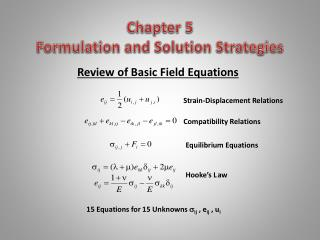# Chapter 5 Formulation and Solution Strategies - PowerPoint PPT PresentationDownload PresentationChapter 5 Formulation and Solution Strategies

Chapter 5 Formulation and Solution StrategiesDownload Presentation## Chapter 5 Formulation and Solution Strategies

- - - - - - - - - - - - - - - - - - - - - - - - - - - E N D - - - - - - - - - - - - - - - - - - - - - - - - - - -
##### Presentation Transcript

1. Review of Basic Field Equations Chapter 5 Formulation and Solution Strategies Strain-Displacement Relations Compatibility Relations Equilibrium Equations Hooke’s L aw 15 Equations for 15 Unknowns ij , eij , ui

2. S S St Su R R R u Boundary Conditions T(n) S = St + Su Traction Conditions Displacement Conditions Mixed Conditions Rigid-Smooth Boundary Condition Allows Specification of Both Traction and Displacement But Only in Orthogonal Directions Symmetry Line y x

3. y xy x r y r  r xy x  y r x r  Boundary Conditions on Coordinate SurfacesOn Coordinate Surfaces the Traction Vector Reduces to Simply Particular Stress Components (Cartesian Coordinate Boundaries) (Polar Coordinate Boundaries)

4. Boundary Conditions on General SurfacesOn General Non-Coordinate Surfaces, Traction Vector Will Not Reduce to Individual Stress Components and General Traction Vector Form Must Be Used Two-Dimensional Example

5. Example Boundary Conditions

6. Interface Boundary Conditions

7. S S St Su R R R u Fundamental Problem Classifications Problem 1 (Traction Problem) Determine the distribution of displacements, strains and stresses in the interior of an elastic body in equilibrium when body forces are given and the distribution of the tractions are prescribed over the surface of the body, T(n) Problem 2 (Displacement Problem) Determine the distribution of displacements, strains and stresses in the interior of an elastic body in equilibrium when body forces are given and the distribution of the displacements are prescribed over the surface of the body. Problem 3 (Mixed Problem) Determine the distribution of displacements, strains and stresses in the interior of an elastic body in equilibrium when body forces are given and the distribution of the tractions are prescribed as per (5.2.1) over the surface St and the distribution of the displacements are prescribed as per (5.2.2) over the surface Su of the body (see Figure 5.1).

8. Stress FormulationEliminate Displacements and Strains from Fundamental Field Equation Set(Zero Body Force Case) Compatibility in Terms of Stress: Beltrami-Michell Compatibility Equations Equilibrium Equations 6 Equations for 6 Unknown Stresses

9. Displacement FormulationEliminate Stresses and Strains from Fundamental Field Equation Set(Zero Body Force Case) Equilibrium Equations in Terms of Displacements:Navier’s/Lame’s Equations 3 Equations for 3 Unknown Displacements

10. General Field Equation System (15 Equations, 15 Unknowns:) Displacement Formulation (3 Equations, 3 Unknowns: ui) Stress Formulation (6 Equations, 6 Unknowns:) Summary of Reduction of Fundamental Elasticity Field Equation Set

11. Principle of Superposition

12. Saint-Venant’s Principle The stress, strain and displacement fields due to two different statically equivalent force distributions on parts of the body far away from the loading points are approximately the same • Boundary loading T(n)would produce detailed and characteristic effects only in vicinity of S*. Away from S*stresses would generally depend more on resultantFR of tractions rather than on exact distribution Stresses approximately the same

13. General Solution Strategies Used to Solve Elasticity Field Equations Direct Method - Solution of field equations by direct integration. Boundary conditions are satisfied exactly. Method normally encounters significant mathematical difficulties thus limiting its application to problems with simple geometry. Inverse Method - Displacements or stresses are selected that satisfy field equations. A search is then conducted to identify a specific problem that would be solved by this solution field. This amounts to determine appropriate problem geometry, boundary conditions and body forces that would enable the solution to satisfy all conditions on the problem. It is sometimes difficult to construct solutions to a specific problem of practical interest. Semi-Inverse Method - Part of displacement and/or stress field is specified, while the other remaining portion is determined by the fundamental field equations (normally using direct integration) and the boundary conditions. It is often the case that constructing appropriate displacement and/or stress solution fields can be guided by approximate strength of materials theory. The usefulness of this approach is greatly enhanced by employing Saint-Venant’s principle, whereby a complicated boundary condition can be replaced by a simpler statically equivalent distribution.

14. Mathematical Techniques Used to Solve Elasticity Field Equations Analytical Solution Procedures - Power Series Method - Fourier Method - Integral Transform Method - Complex Variable Method Approximate Solution Procedures - Ritz Method Numerical Solution Procedures - Finite Difference Method (FDM) - Finite Element Method (FEM) - Boundary Element Method (BEM)